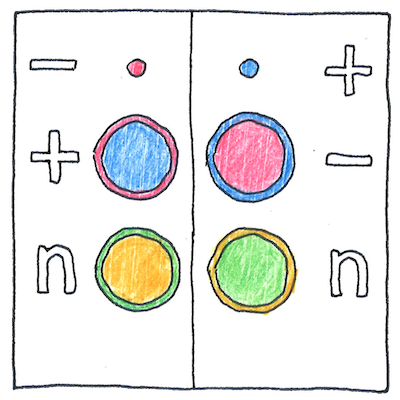1932

The book of science

Tom Sharp

 Paul Dirac, Carl David Anderson physics

Antimatter

• Paul Dirac developed a relativistic wave equation
• that describes the behavior of electrons
• and predicted the existence of antimatter.
• Carl Anderson discovered the positron,
• the antimatter particle corresponding to the electron,
• recorded in a cloud chamber from a cosmic ray collision.
• When particles of antimatter and matter interact
• the mass of both particles is converted into energy
• according to Einstein’s equation, its mass multiplied
• by the square of the speed of light.

Asymmetry

• Small amounts of antimatter are created throughout the universe
• by high-energy cosmic rays, but it is immediately
• annihilated by an equal amount of ordinary matter on contact.
• If the creation of matter and antimatter were equally likely
• why is antimatter so scarce in this universe?
• Otherwise, why is it not equally likely? One can only say
• that if it were equally likely then matter and antimatter
• would have annihilated each other long before now.

Untestable hypotheses

• Here I deny
• the necessity
• and sufficiency
• of all our means
• of seeing—
• Many things
• exist that we
• cannot see
• or, in seeing,
• do not know.

The current theory is that matter and antimatter must be created in equal amounts, in pairs; however, in our universe matter vastly overwhelms antimatter. Was there a slight imbalance in the amounts created? Does antimatter decay faster than matter? Did all the antimatter get pushed to distant galaxies or sucked into a parallel universe?

Annihilation of an electron and a positron at rest produces two gamma ray photons; that is, annihilation produces pure energy; however, other types of matter-antimatter collisions are more complex. Annihilation of a proton and an antiproton produces mesons that decay into photons, positrons, electrons, and neutrinos.

See also in The book of science: